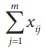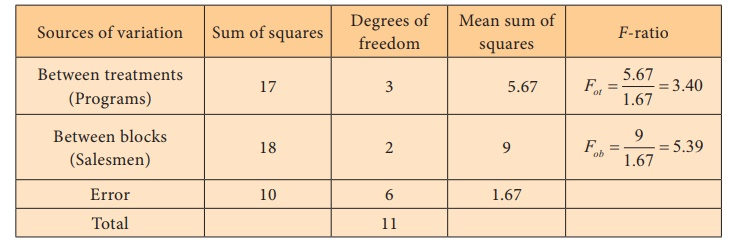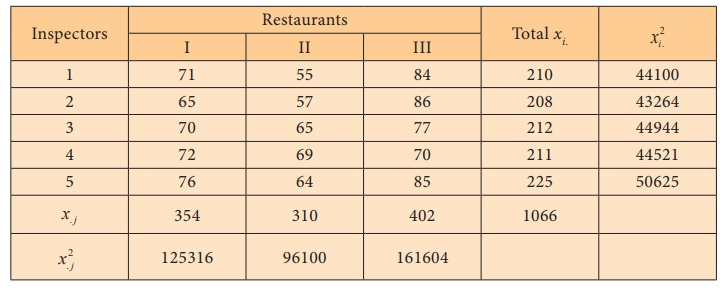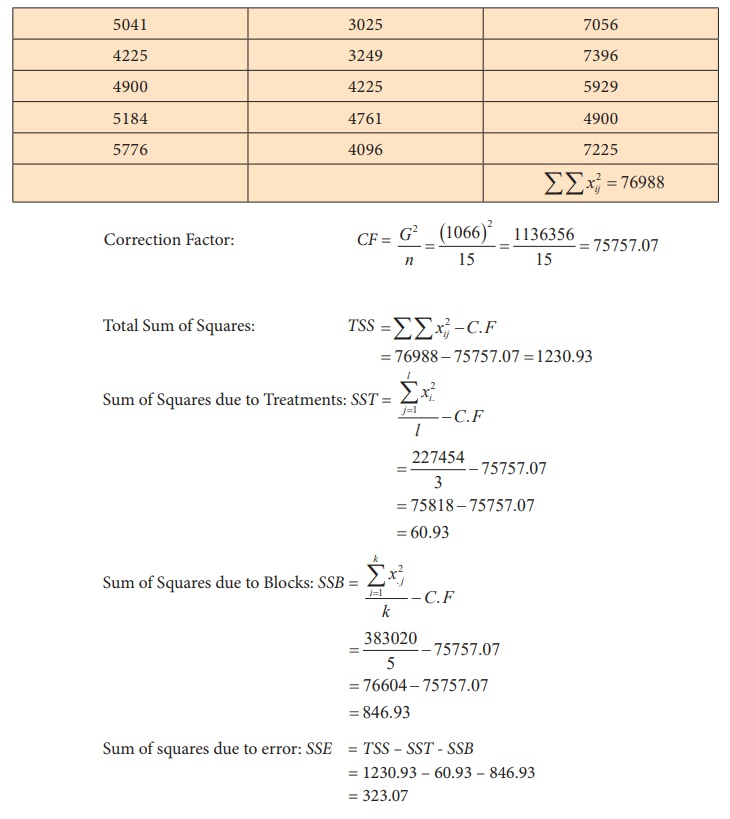Home | | Statistics 12th Std | Two Way ANOVA

# Two Way ANOVA

Two Way ANOVA: Test Procedure, Merits and Demerits, Example Solved Problems | Analysis of Variance | Statistics

TWO-WAY ANOVA

In two-way ANOVA a study variable is compared over three or more groups, controlling for another variable. The grouping is taken as one factor and the control is taken as another factor. The grouping factor is usually known as Treatment. The control factor is usually called Block. The accuracy of the test in two -way ANOVA is considerably higher than that of the one-way ANOVA, as the additional factor, block is used to reduce the error variance.

In two-way ANOVA, the data can be represented in the following tabular form.

BlocksWe use the following notations.

xij - ith treatment value from the jth block, i = 1,2, ..., k; j = 1,2, ..., m.

The ith treatment total - xi, i 1, 2, ..., k

The jth block total - x . j xij , j 1, 2, ..., m

Note that, k × m = n, where m = number of blocks, and k = number of treatments (groups) and n is the total number of observations in the study.

The total variation present in the observations xij can be split into the following three components:

(i) The variation between treatments (groups)

(ii) The variation between blocks.

(ii) The variation inherent within a particular setting or combination of treatment and block.

1. Test Procedure

Steps involved in two-way ANOVA are:

Step 1 : In two-way ANOVA we have two pairs of hypotheses, one for treatments and one for the blocks.

Framing Hypotheses

Null Hypotheses

H01: There is no significant difference among the population means of different groups (Treatments)

H02: There is no significant difference among the population means of different Blocks

Alternative Hypotheses

H11: Atleast one pair of treatment means differs significantly

H12: Atleast one pair of block means differs significantly

Step 2 : Data is presented in a rectangular table form as described in the previous section.

Step 3 : Level of significance α.

Step 4 : Test Statistic

F0t (treatments) = MST / MSE

F0b (block) = MSB / MSE

To find the test statistic we have to find the following intermediate values.v) Sum of Squares due to Error: SSE = TSS-SST-SSB

vi) Degrees of freedomvii) Mean Sum of SquaresStep 5 : Calculation of the Test Statistic

ANOVA Table (two-way)Step 6 : Critical values

Critical value for treatments = f(k-1,(m-1)(k-1)),α

Critical value for blocks = f(m-1, (m-1)(k-1)),α

Step 7 : Decision

For Treatments: If the calculated F0t value is greater than the corresponding critical value, then we reject the null hypothesis and conclude that there is significant difference among the treatment means, in atleast one pair.

For Blocks: If the calculated F0b value is greater than the corresponding critical value, then we reject the null hypothesis and conclude that there is significant difference among the block means, in at least one pair.

2. Merits and Demerits of two-way ANOVA

Merits

·              Any number of blocks and treatments can be used.

·              Number of units in each block should be equal.

·              It is the most used design in view of the smaller total sample size since we are studying two variable at a time.

Demerits

·              If the number of treatments is large enough, then it becomes difficult to maintain the homogeneity of the blocks.

·              If there is a missing value, it cannot be ignored. It has to be replaced with some function of the existing values and certain adjustments have to be made in the analysis. This makes the analysis slightly complex.

Comparison between one-way ANOVA and two-way ANOVAExample 3.6

A reputed marketing agency in India has three different training programs for its salesmen. The three programs are Method – A, B, C. To assess the success of the programs, 4 salesmen from each of the programs were sent to the field. Their performances in terms of sales are given in the following table.Test whether there is significant difference among methods and among salesmen.

Solution:

Step 1 : Hypotheses

Null Hypotheses: H01 : μM1= μM2 = μM3 (for treatments)

That is, there is no significant difference among the three programs in their mean sales.

H02 : μS1 = μS2 = μS3 = μS4 (for blocks)

Alternative Hypotheses:

H11 : At least one average is different from the other, among the three programs.

H12 : At least one average is different from the other, among the four salesmen.

Step 2 : DataStep 3 : Level of significance α = 5%

Step 4 : Test StatisticStep-5 : Calculation of the Test StatisticANOVA Table (two-way)Step 6 : Critical values

f(3, 6),0.05 = 4.7571 (for treatments)

f(2, 6),0.05 = 5.1456 (for blocks)

Step 7 : Decision

(i) Calculated F0t = 3.40 < f(3, 6),0.05 = 4.7571, the null hypothesis is not rejected and we conclude that there is significant difference in the mean sales among the three programs.

(ii) Calculate F0b = 5.39 > f(2, 6),0.05 = 5.1456, the null hypothesis is rejected and conclude that there does not exist significant difference in the mean sales among the four salesmen.

Example 3.7

The illness caused by a virus in a city concerning some restaurant inspectors is not consistent with their evaluations of cleanliness of restaurants. In order to investigate this possibility, the director has five restaurant inspectors to grade the cleanliness of three restaurants. The results are shown below.Carry out two-way ANOVA at 5% level of significance.

Solution:

Step 1 :

Null hypotheses

H0I : µ1 = µ 2 = µ 3 = µ 4 = µ5 (For inspectors - Treatments)

That is, there is no significant difference among the five inspectors over their mean cleanliness scores

H0R : μI = μII = μIII (For restaurants - Blocks)

That is, there is no significant difference among the three restaurants over their mean cleanliness scores

Alternative Hypotheses

H1I: At least one mean is different from the other among the Inspectors

H1R: At least one mean is different from the other among the Restaurants.

Step 2 : DataStep 3 : Level of significance α = 5%

Step 4 : Test Statistic

For inspectors: F0a (treatments) = = MST / MSE

For restaurants: F0b (blocks) = MSE / MSB

Step-5 : Calculation of the Test StatisticSquaresANOVA Table (two-way)Step 6 : Critical values

f(4, 8),0.05 = 3.838 (for inspectors)

f(2, 8),0.05 = 4.459 (for restaurants)

Step 7 : Decision

(i) As F0I = 0.377 < f(4, 8),0.05 = 3.838, the null hypothesis is not rejected and we conclude that there is no significant difference among the mean cleanliness scores of inspectors.

(ii) As F0R = 10.49 > f(2, 8),0.05 = 4.459, the null hypothesis is rejected and we conclude that there exists significant difference in atleast one pair of restaurants over their mean cleanliness scores.

Tags : Test Procedure, Merits and Demerits, Example Solved Problems | Analysis of Variance | Statistics , 12th Statistics : Chapter 3 : Tests Based on Sampling Distributions II
Study Material, Lecturing Notes, Assignment, Reference, Wiki description explanation, brief detail
12th Statistics : Chapter 3 : Tests Based on Sampling Distributions II : Two Way ANOVA | Test Procedure, Merits and Demerits, Example Solved Problems | Analysis of Variance | Statistics